Transaction

Overiew
Transaction is encoded data stracture that value transfer between participants in Bitcoin sistem.
Individual transaction add into blockchain as global ledger.
Anyone can see each transaction in the blockchain.
Basic component of transaction is the unused transaction output(UTXO).
UTXO is chunks of undividable Bitcoin locked to a specific owner.

Transaction life cycle
Transaction generate→　Transaction signature　→　Broadcast to network →　Network verification →　Propagation to network →　Mining node verfication →　Recode to blockchain

Transaction construction
The transaction contains several fields as shown in the following transaction structure.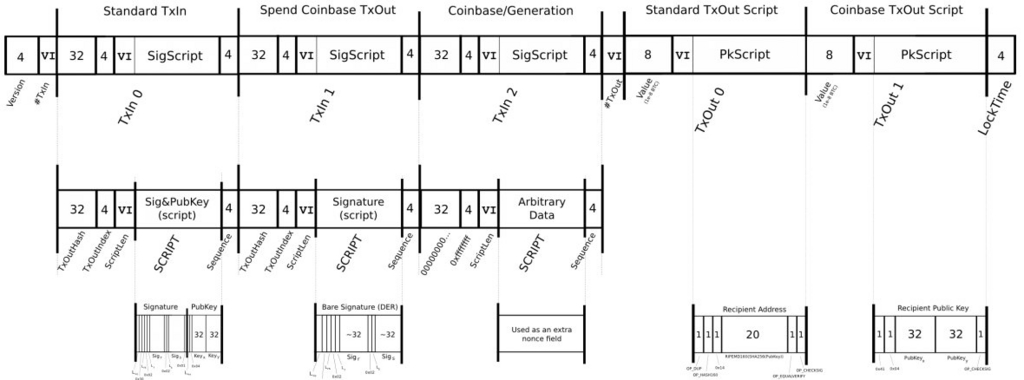Version(4byte):
→Specifies which rules this transaction follows

Input values(1byte):
→How many input are included

Input
Previous block hash(32byte):
→Pointer to the transaction containing the UTXO to be spent

Previous index(4byte):
→The index number of the UTXO to be spent; first one is 0

Script bytes length(1byte):
→Unlocking-Script length in bytes

scriptSig:
→A script that fulfills the conditions of the UTXO locking script

Terminal symbols(4byte):
→Currently disabled Tx-replacement feature, set to "0xFFFFFFFF"

Output values(1byte):
→How many output are included

Output
Amount invested(8byte):
→Bitcoin value in satoshis

Script byte length(1byte):
→Locking-Script length in bytes

ScriptPubkey:
→A script defining the conditions needed to spend the output

Lock time(4byte):
→A Unix timestampn or block number

Locktime = 0: Propagated immediately

Lock time ≠ 0> 500,000,000: Block height (meaning that this transaction is not included in the block chain in the block before this)

Lock time ≠ 0 <500,000,000: Unix time (meaning this transaction is not valid before this time)

Transaction type
Bitcoin uses a scripting system for transactions.
Forth-like, Script is simple, stack-based, and processed from left to right.
To prevent attacks on the Bitcoin network by not allowing complicated processing.
There are five standard transaction scripts for Bitcoin.

①pay-to-public-key-hash(P2PKH)
The scripte is used vast majority of transactions on the bitcoin network.The P2PKH designate Bitcoin address as payee.An output locked by a P2PKH script can be unlocked (spent) by presenting a public key and a digital signature created by the corresponding private key.

unlocking script(scriptSig)

```<Signature><Public Key>
```

unlocking script exemple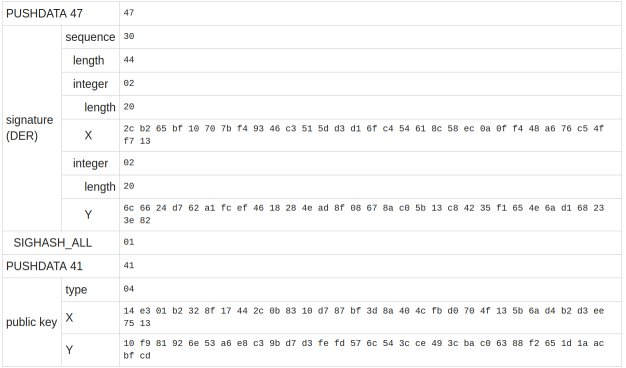locking script(scriptPublicKey)

```OP_DUP OP_HASH160<public Key Hash> OP_EQUAL OP_CHECKSIG
```

locking script exemple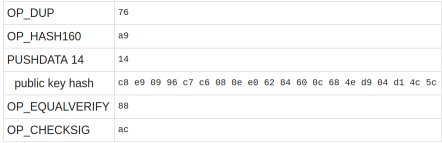unlocking script + locing script

```<Signature><Public Key> OP_DUP OP_HASH160<public Key Hash> OP_EQUAL OP_CHECKSIG
```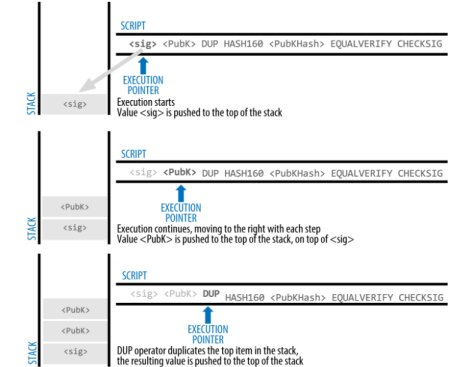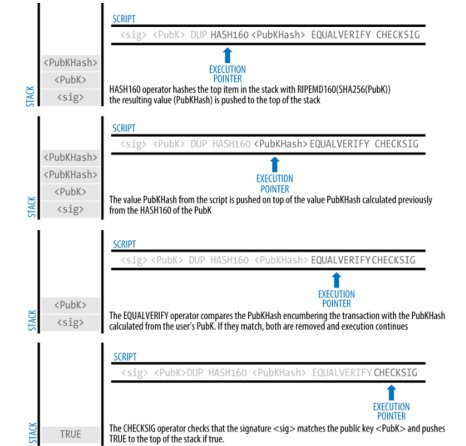②pay-to-public-key(P2PK)
P2PK is a simplified form of P2PKH,P2PK is not safer than P2PKH and generally don't use now.

locking script

`<Public key A><OP_CHECKSIG>`

unlocking script

`<Signature from Private Key A>`

unlocking script + locking script

`<Signature from Private Key A><Public key A>OP_CHECKSIG`

③pay-to-multi-signature(P2MS)
Multi-signature scripts must set N public keys and recorded in the script and at least M number of signatures must provide when release. This is also known as an M-of-N scheme, where N is the total number of keys and M is the threshold of signatures required for validation.

locking script

`2<Public Key A><Public Key B><Public Key C>3 OP_CHECKMULTISIG`

unlocking script

`OP_0<Signature B><Signature C> `

unlocking script + locking script

`OP_0<Signature B><Signature C>2<Public Key A><Public Key B><Public Key C>3 OP_CHECKMULTISIG`

④data output(OP_RETURN)
OP_RETURN can add 80 bytes of data unrelated to payment to the transaction.OP_RETURN don't have "unlock script" for use to output.This will not be an available UTXO,output is assigned with 0BTE.If Script verifcation software find to OP_RETURN,Stops execution of the verification script immediately and invalidates the transaction

⑤pay-to-script-hash(P2SH)
The Multisig use multiple PubKey at the time of remittance ,Locking Script has a problem that the amount of data becomes large.In P2SH, PubKey data is separated from the Locking Script at the time of payment,and that Public key is included in "Redeem Script" and the problem was solved by referring to data.

When P2MS used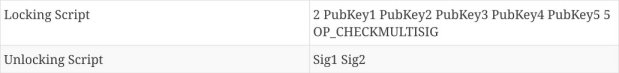When P2SH used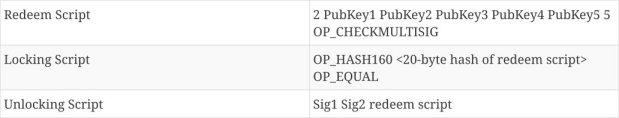P2MS scriptActually P2MS data is long like this!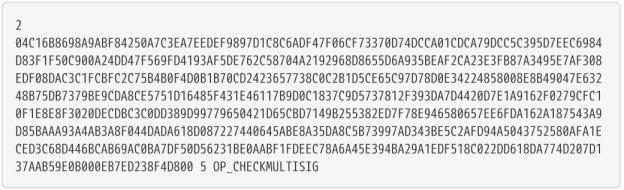Even such a long script become short when expressing with SHA 160(20byte)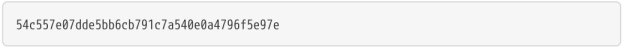In other word,P2SH can be expressed simply as follows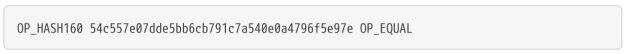When using UTXO created with P2SH
Redeem Script is checked against Locking Script whether this hash matches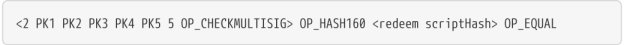Unlocking Script will be executed to release of Redeem Script when Redeem Script matches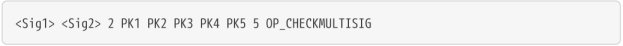※P2SH address is encoded Hash value of Redeem script,Version Prefix is "5" and P2SH address is beginning "3"

Transaction Signature
There are various methods among digital signatures,but Elliptic Curve Digital Signature Algorithm (ECDSA) is used in Bitcoin.The ECDSA offers Digital Signature Algorithm (DSA) which is used ECC.This cryptographic algorithm ensure that funds can only be spent by their rightful owners.

ECDSA
When Alice send message(m) and signature(u, v) to Bob who can be verified data has not been tampered by third parties.

1.In advance Alice generate private key(d) and publishing public key(Q)

`Q = d * G　...①　※Prime numbers(I) and Base point(G) known to Bob`

2.Generate rundam numbers(r),and r times moved point R(coordinate xU,yU) calculate from base point(G)

`r * G = R =(xU, yU)`

3.Find the hash value H of message m

4.Calculate signature value(u, v)

```u = xU　( mod l)
v = (H + u * d) / r　(mod l) ...②　※The remainder u dividing xU to l(prime number)```

5.The signature(u, v) send to Bob

6.Point P calcurated using public key(Q) and parameter(prime number l, base point G) and signature value(u, v)

`P  = (xV, yV)　= H/ v*G + u /v*Q　...③`

7.If "xV" and "xU mod l" are equal,they has not been tampered

Why is this confirming the signature?

1.Let's verify using ③ formula

```P =  (xV, yV)　= H/ v*G + u /v*Q

From ①

= H/v * G + u/v * dG

= 1/v (H + ud)G

From ②

= r / (H + ud)  * (H + ud)G

=rG = R```

When the signature value (u, v), the hash value H, and the public key Q are correct.Since the point P and the point R are equal, the signature value (u) of the point R is equal to the x coordinate (xV) of the point P.Therefore, the signature is correct.

Transaction signature flow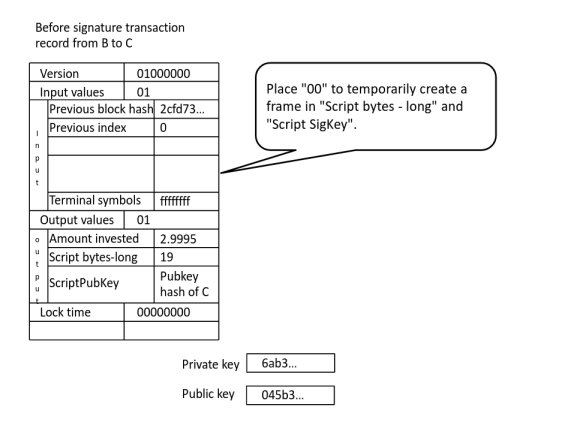```priv = sha256('some big long brainwallet password')
pub = privtopub(priv)
outs = [{'value': 90000, 'address': '16iw1MQ1sy1DtRPYw3ao1bCamoyBJtRB4t'}]
tx = mktx(h,outs)
```

→The history() retrieves all transaction outputs (TXO) that have been "received" by the specified bitcoin address. This includes both unspent and spent TXOs.if marked with "spend",meaning that have been spent.

tx = mktx(h,outs)
→The tx create frame of transaction that include as shown in the figure above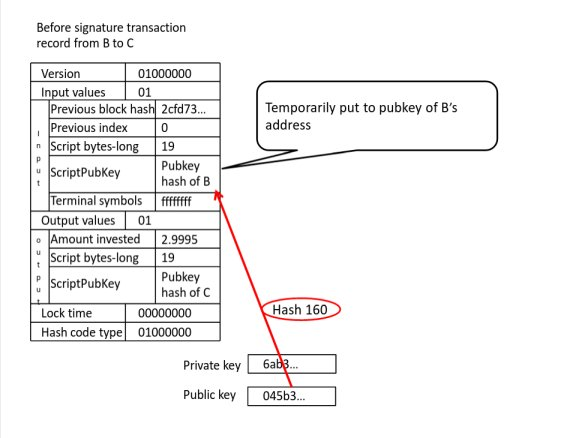```def sign(tx, i, priv, hashcode=SIGHASH_ALL):
pub = privkey_to_pubkey(priv)
#temporary staging to Public key hash
#signature_from:add scriptSig + previous output index
#76a914...88ac...(P2PKH)
signing_tx = signature_form(tx, int(i), '76a914' + b58check_to_hex(address) + '88ac', hashcode)
```

def sign()
→This function generate signatur use private key

signing_tx = signature_form(tx, int(i),
'76a914' + b58check_to_hex(address) + '88ac', hashcode)

→A normal sendtoaddress transaction will start with 76a914 and end with 88ac.and 76a914 means "OP_DUP OP_HASH160 PUSH_20BYTES" and 88ac is "OP_EQUALVERIFY OP_CHECKSIG".
→Temporarily put to ScriptPubKey and Script bytes-long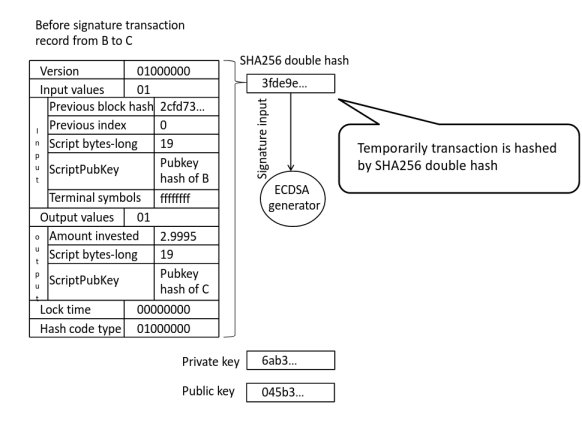```def deterministic_generate_k(msghash, priv):
v = b'\x01' * 32
k = b'\x00' * 32
priv = encode_privkey(priv, 'bin')
msghash = encode(hash_to_int(msghash), 256, 32)
k = hmac.new(k, v+b'\x00'+priv+msghash, hashlib.sha256).digest()
v = hmac.new(k, v, hashlib.sha256).digest()
k = hmac.new(k, v+b'\x01'+priv+msghash, hashlib.sha256).digest()
v = hmac.new(k, v, hashlib.sha256).digest()
return decode(hmac.new(k, v, hashlib.sha256).digest(), 256)
```

def ecdsa_raw_sign(msghash, priv)
→RFC6979 is a deterministic digital signature generation procedure.If ECDSA implementation bad,private keys have leaked.Deterministic signatures retain the cryptographic security features associated with digital signatures but can be more easily implemented in various environments, since they do not need access to a source of high-quality randomness.Therefore, it must implement deterministic signature schemes such as the scheme described in RFC6979.

※Section 3.2 of RFC6979 is described the process of generating nonce.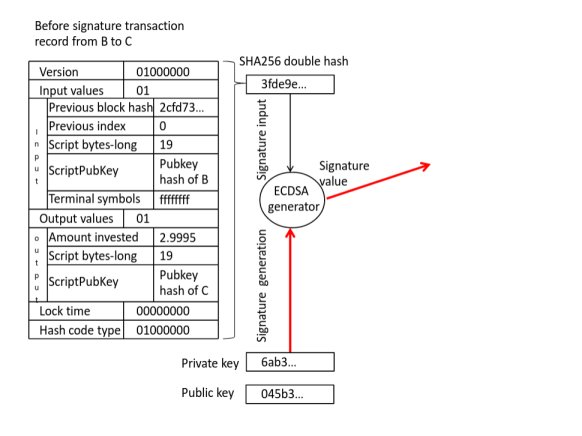```def ecdsa_raw_sign(msghash, priv):
z = hash_to_int(msghash)
k = deterministic_generate_k(msghash, priv)
r, y = fast_multiply(G, k)
s = (z + r*decode_privkey(priv)) * inv(k, N) % N
v = 27+((y % 2) ^ (0 if s * 2 < N else 1))
s = s if s * 2 < N else N - s
return v, r, s
```

r, y = fast_multiply(G, k)
→Use ECC to find the point R
→r * G = R =(xU, yU) ※Point R is r times moved point R
→u = xU(mod l) u is signature value(In above code it is "r")

s = (z + r*decode_privkey(priv)) * inv(k, N) % N
→The other signature value calculated from "v = (H + u * d) / r ( mod l)"

v = 27 +( (y % 2) ^ (0 if s * 2 < N else 1) )
→It returns 27 when V is an even number, and 28 in case of an odd number

s = s if s * 2 < N else N - s
→The number of prime points on the plane is limited to order N.
→N is parameters of the "secp256k1" used in Bitcoin
※N=115,792,089,237,316,195,423,570,985,008,687,907,852,837,564,279,074,904,382,605,163,141,518,161,494,337

```def der_encode_sig(v, r, s):
b1 = str(binascii.hexlify(encode(r, 256)), 'utf-8')
b2 = str(binascii.hexlify(encode(s, 256)), 'utf-8')
left = '02'+encode(len(b1)//2, 16, 2)+b1
right = '02'+encode(len(b2)//2, 16, 2)+b2
return '30'+encode(len(left+right)//2, 16, 2)+left+right

def ecdsa_tx_sign(tx, priv, hashcode=SIGHASH_ALL):
rawsig = ecdsa_raw_sign(binascii.unhexlify(txhash(tx, hashcode)), priv)
return der_encode_sig(*rawsig)+encode(hashcode, 16, 2)
```

def der_encode_sig(v, r, s)
→DER encoded signature has the following form
0x30 b1 0x02 b2 r 0x02 b3 s
DER is a set of rules for transforming a data structure into a sequence of bytes, and back.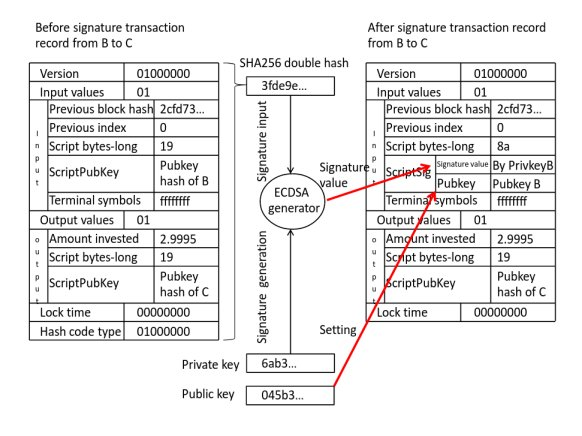```def sign(tx, i, priv, hashcode=SIGHASH_ALL):
pub = privkey_to_pubkey(priv)
signing_tx = signature_form(tx, int(i),
'76a914' + b58check_to_hex(address) + '88ac', hashcode)
sig = ecdsa_tx_sign(signing_tx, priv, hashcode)
txobj = deserialize(tx)
txobj["ins"][int(i)]["script"] = serialize_script([sig, pub])
return serialize(txobj)
```

txobj = deserialize(tx)
→Restore the signed tx to the original data format

txobj["ins"][int(i)]["script"] = serialize_script([sig, pub])
→The signature value and public key put in ScriptSig of input